# Test: Power Electronics- 5

## 10 Questions MCQ Test GATE Electrical Engineering (EE) 2023 Mock Test Series | Test: Power Electronics- 5

Description
Attempt Test: Power Electronics- 5 | 10 questions in 30 minutes | Mock test for Electrical Engineering (EE) preparation | Free important questions MCQ to study GATE Electrical Engineering (EE) 2023 Mock Test Series for Electrical Engineering (EE) Exam | Download free PDF with solutions
*Answer can only contain numeric values
QUESTION: 1

### A 3 ϕ 180°-mode bridge inverter has star-connected load of R = 8 Ω and L-30 mH. The inverter is fed from 220 V dc and its output frequency is 50 Hz. Find the RMS value of 3rd harmonic of line current (in A)

Solution: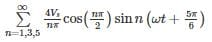All triplen harmonics are absent from the line voltage

*Answer can only contain numeric values
QUESTION: 2

### Find the commutation time (in ms) for a 3 phase full converter (RLE load) at firing angle 30°. (Answer should be in ms)

Solution: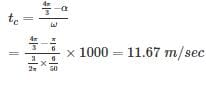QUESTION: 3

### A 6-pulse thyristor bridge is connected to a balanced 50 Hz , 3 - ϕ ac source. Assuming the dc o/p current of the rectifier is constant, the lowest frequency harmonic component of the source line current is

Solution:

Ripple frequency = 5 x 50 = 250 Hz. Hence the least frequency component is of 250 Hz

QUESTION: 4

The overlap angle of a phase controlled converter will increase if

1. The firing angle increases
2. The frequency of supply increases
3. The supply voltage increases

Among the above statements

Solution:

With increase in frequency the effective reactance at source increases which increase overlap angle. If supply voltage increases current will take more time to build up through source inductance, hence μ increases.  If firing angle increases to maintain constant average current overlap angle will decrease. (observe by putting α=0,30,60 etc)

QUESTION: 5

A separately excited DC motor fed from 3-phase semi converter develop a full load torque at 1500 rpm when firing angle is 60°. The armature taking 50 A at 400 V DC and having the resistance of 0.4 Ω, then supply voltage per phase will be ________

Solution:

Concept: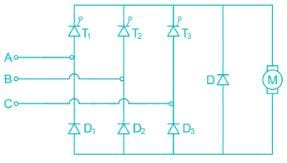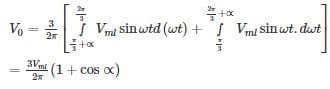Calculation:

We know that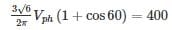VPh = 228 V

*Answer can only contain numeric values
QUESTION: 6

A 3 ϕ 180°-mode bridge inverter has star-connected load of R = 8 Ω and L-30 mH. The inverter is fed from 220 V dc and its output frequency is 50 Hz. Find the R.M.S value of 1st harmonic current (in A)

Solution:

Vs = 220 V, R = 8 Ω, ωL = 2π × 50 × 30 × 10-3 = 9.42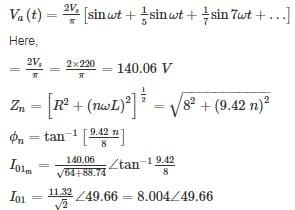*Answer can only contain numeric values
QUESTION: 7

A 3-ϕ, 3 pulse converter, fed from delta star transformer, is connected to a load requiring ripple free current. A freewheeling diode is connected across the load. Find the R.M.S value of thyristor current (in A) for firing angle 60°. (Take Vmp = 200 V)

Solution:

Average value of the output voltage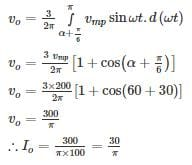R.M.S value of thyristor current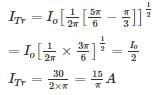QUESTION: 8

A 240 V battery is being charged through a three phase diode bridge rectifier. The rectifier is fed by a three – phase 230 V. 50 Hz source current limiting resistance in series with battery is 8Ω and load current is assumed to be ripple free. The value of average battery charge current is –

Solution:

Vm = √2 × 230 V
Average output voltage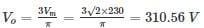let the battery emf is E and average charging current is Io then,
VO = E + IO R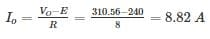*Answer can only contain numeric values
QUESTION: 9

A solar cell of 400 V is feeding power to an ac supply of 440 V, 50 Hz through a 3 – phase fully controlled bridge converter. A large inductance is connected in the dc circuit to maintain the dc current at 12 A. If the solar cell resistance is 1 Ω, then each thyristor will be reverse biased for a period of – (in degrees)

Solution:

Rsolar = 1 Ω, Io = 12 A

Vs = 400 – 12 (1) = 388 V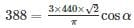⇒ α = 49.23°

So each thyristor will reverse biased for

180° - 49.23 = 130.77°

QUESTION: 10

A three phase fully controlled bridge converter is feeding a load drawing a constant and ripple free load current of 20 A at a firing angle of 60°. The approximate total harmonic distortion (% THD) and the rms value of fundamental component of input current will respectively

Solution:

Total rms current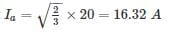Fundamental current Ia1 = 0.78 × 20 = 15.6A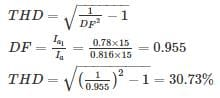Use Code STAYHOME200 and get INR 200 additional OFF Use Coupon Code